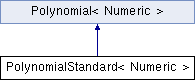clipper
PolynomialStandard< Numeric > Class Template Reference

Represents a polynomial in standard monomial base. More...

Inheritance diagram for PolynomialStandard< Numeric >:List of all members.

## Public Member Functions

PolynomialStandard ()
PolynomialStandard (const std::vector< Numeric > &coefficient)
PolynomialStandard (Numeric factor, const std::vector< Numeric > &roots)
Construct polynomial from roots and a scaling multiplier.
unsigned int degree () const
Return degree of the polynomial.
Numeric coefficient (unsigned int i) const
Return a monomial coefficient.
std::string toString (const std::string &X="X") const
Return polynomial as latex math string.
std::string toStringPlot () const
Return polynomial as string for plotting with gnuplot.
Numeric evaluate (Numeric X) const
Calculate polynomial's value at the position X using Horner's schema.
std::vector< Numeric > findRoots () const
Calculate all real roots for a quadratic or cubic polynomial using the p-q- or Cardano's formulas.
PolynomialStandard scale (Numeric alpha, Numeric beta) const
Apply the linear transformation "X * (beta - alpha) + alpha" to the polynomial.
class PolynomialBezier< Numeric > toBezier () const
Convert the polynomial from standard base to the Bernstein-Bezier basis in the unit interval.

## Private Attributes

std::vector< Numeric > m_coefficient
Coefficients of the polynomial. Degree is m_coefficients.size()-1.

## Detailed Description

### template<typename Numeric> class PolynomialStandard< Numeric >

Represents a polynomial in standard monomial base.

Definition at line 786 of file clipper.cc.

## Constructor & Destructor Documentation

template<typename Numeric>
 PolynomialStandard< Numeric >::PolynomialStandard ( ) ` [inline]`

Definition at line 794 of file clipper.cc.

template<typename Numeric>
 PolynomialStandard< Numeric >::PolynomialStandard ( const std::vector< Numeric > & coefficient ) ` [inline, explicit]`

Definition at line 798 of file clipper.cc.

template<typename Numeric>
 PolynomialStandard< Numeric >::PolynomialStandard ( Numeric factor, const std::vector< Numeric > & roots ) ` [inline]`

Construct polynomial from roots and a scaling multiplier.

Definition at line 804 of file clipper.cc.

## Member Function Documentation

template<typename Numeric>
 Numeric PolynomialStandard< Numeric >::coefficient ( unsigned int i ) const` [inline]`

Return a monomial coefficient.

Definition at line 858 of file clipper.cc.

template<typename Numeric>
 unsigned int PolynomialStandard< Numeric >::degree ( ) const` [inline]`

Return degree of the polynomial.

Definition at line 852 of file clipper.cc.

template<typename Numeric>
 Numeric PolynomialStandard< Numeric >::evaluate ( Numeric X ) const` [inline]`

Calculate polynomial's value at the position X using Horner's schema.

Definition at line 913 of file clipper.cc.

template<typename Numeric>
 std::vector PolynomialStandard< Numeric >::findRoots ( ) const` [inline]`

Calculate all real roots for a quadratic or cubic polynomial using the p-q- or Cardano's formulas.

Definition at line 926 of file clipper.cc.

template<typename Numeric>
 PolynomialStandard PolynomialStandard< Numeric >::scale ( Numeric alpha, Numeric beta ) const` [inline]`

Apply the linear transformation "X * (beta - alpha) + alpha" to the polynomial.

This remaps the interval [alpha,beta] to [0,1].

Definition at line 1033 of file clipper.cc.

template<typename Numeric >
 PolynomialBezier< Numeric > PolynomialStandard< Numeric >::toBezier ( ) const

Convert the polynomial from standard base to the Bernstein-Bezier basis in the unit interval.

Convert the polynomial from monomial representation to the Bernstein-Bezier basis in the unit interval.

Use scale() to transform the coefficients before applying this base conversion.

Definition at line 1429 of file clipper.cc.

template<typename Numeric>
 std::string PolynomialStandard< Numeric >::toString ( const std::string & X = `"X"` ) const` [inline]`

Return polynomial as latex math string.

Definition at line 865 of file clipper.cc.

template<typename Numeric>
 std::string PolynomialStandard< Numeric >::toStringPlot ( ) const` [inline]`

Return polynomial as string for plotting with gnuplot.

Definition at line 890 of file clipper.cc.

## Member Data Documentation

template<typename Numeric>
 std::vector PolynomialStandard< Numeric >::m_coefficient` [private]`

Coefficients of the polynomial. Degree is m_coefficients.size()-1.

Definition at line 791 of file clipper.cc.

The documentation for this class was generated from the following file: